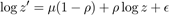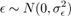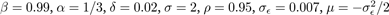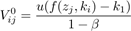# We Helped With This Macroeconomics Assignment: Have A Similar One?

SOLVEDCategory Economics Macroeconomics Graduate Solved Best Macroeconomics Assignment Help

## Assignment Description

Assignment 6

Solving an RBC model using Discrete State-Space

Consider a neoclassical growth model with aggregate shocks. Use the following functional assumptions: Per-period utility is represented by u(c) = (c1−σ −1)/(1−σ) and production function is zF(k,1) = zkα. The representative agent discounts future at rate 0 < β < 1 and capital depreciates at rate 0 < δ < 1. The aggregate shock z is estimated to follow the following AR(1) processwhereFor the entire assignment, you will use a discretized version of this process.

Use the following parameter values:,(Stopping rule).

(a)     Use the Euler equation from a version of this model without uncertainty (z = 1 for all periods) to find the steady-state capital stock kss numerically. Use n = 1000 equidistant points between 0.85kss and 1.15kss for the discretized state-space K = {k1,...,kn}.

(b)     Use Tauchen’s method to discretize the continuous AR(1) process into m = 17 discrete states Z = {z1,...,zm}. Use 3 standard deviations on each side of the unconditional mean of logz for the interval.

(Do not forget to convert logz grids into z grids at the end.)

(c)     Usefor each i,j

as the initial guess for the value function, where f(z,k) = zF(k,1) + (1 − δ)k.

(d)     Solve the model using any of the discrete state method you learned so far. It is recommended that you use Howard’s Improvement algorithm. You can use very high Nh (e.g. Nh=100) to speed up the computation. (Warning: This will take a long time if you do not use any acceleration methods.)

(e)     On the same graph, plot the policy function for each value of z separately. (g(k,z1),g(k,z2),...,g(k,zm) on the same graph). Similarly, on a separate graph, plot the value functions for every value of z.

(f)      Starting from initial value z = z9, using the Markov matrix you constructed in part b, simulate timeseries for z. Use 10000 time periods. Using this time-series for z, along with initial capital stock equal to the steady-state level you found in part a (find the grid point that is closest to steady-state capital), simulate time-series for capital stock, investment, consumption and output for 10000 time periods. Graph the last 1000 periods of each time-series in log scale (logkt, logct, logIt) on separate plots.

(g)     Calculate the standard deviation (volatility) of each variable and correlation of each variable with output (all in log scale). Report all the results.

1

Is it free to get my assignment evaluated?

Yes. No hidden fees. You pay for the solution only, and all the explanations about how to run it are included in the price. It takes up to 24 hours to get a quote from an expert. In some cases, we can help you faster if an expert is available, but you should always order in advance to avoid the risks. You can place a new order here.

How much does it cost?

The cost depends on many factors: how far away the deadline is, how hard/big the task is, if it is code only or a report, etc. We try to give rough estimates here, but it is just for orientation (in USD):

 Regular homework \$20 - \$150 Advanced homework \$100 - \$300 Group project or a report \$200 - \$500 Mid-term or final project \$200 - \$800 Live exam help \$100 - \$300 Full thesis \$1000 - \$3000

How do I pay?

Credit card or PayPal. You don't need to create/have a Payal account in order to pay by a credit card. Paypal offers you "buyer's protection" in case of any issues.

Why do I need to pay in advance?

We have no way to request money after we send you the solution. PayPal works as a middleman, which protects you in case of any disputes, so you should feel safe paying using PayPal.

Do you do essays?

No, unless it is a data analysis essay or report. This is because essays are very personal and it is easy to see when they are written by another person. This is not the case with math and programming.

Why there are no discounts?

It is because we don't want to lie - in such services no discount can be set in advance because we set the price knowing that there is a discount. For example, if we wanted to ask for \$100, we could tell that the price is \$200 and because you are special, we can do a 50% discount. It is the way all scam websites operate. We set honest prices instead, so there is no need for fake discounts.

Do you do live tutoring?

No, it is simply not how we operate. How often do you meet a great programmer who is also a great speaker? Rarely. It is why we encourage our experts to write down explanations instead of having a live call. It is often enough to get you started - analyzing and running the solutions is a big part of learning.

What happens if I am not satisfied with the solution?

Another expert will review the task, and if your claim is reasonable - we refund the payment and often block the freelancer from our platform. Because we are so harsh with our experts - the ones working with us are very trustworthy to deliver high-quality assignment solutions on time.

Customer Feedback

"Thanks for explanations after the assignment was already completed... Emily is such a nice tutor! "

Order #13073